RS Aggarwal Solutions for Class 9 Maths Chapter 5 - Congruence of Triangles Ex 5A (5.1)

RS Aggarwal Class 9 RS Aggarwal Solutions for Class 9 MathsChapter 5 - Congruence of Triangles Ex 5A (5.1) Solutions Free PDF

Question 1:

In the adjoining figure, PA $\perp$ AB, QB $\perp$ AB and PA = QB. If PQ intersects AB at O, show that O id the mid-point of AB as well as that of PQ.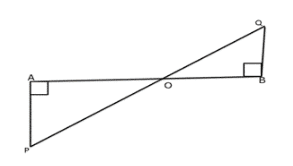Solution:

Given: PA $\perp$ AB, QB $\perp$ AB, and PA = QB

To prove: AO = OB and PO = OQ

Proof: In $\Delta$APO and $\Delta$BPO,

$\angle$PAO = $\angle$QBO = 90o [Given]

PA = QB [Given]

$\angle$PAO = $\angle$QBO [Since PA $\perp$ AB, and QB $\perp$ AB, PA || QB, and thus PQ is a transversal, therefore, alternate angles are equal]

So, by Angle-Side-Angle criterion of congruence, we have

$\Delta$APO $\cong$ $\Delta$BPO

$\Rightarrow$ AO = OB and PO = OQ [Since corresponding parts of congruent triangles are equal]

Thus, we have O is the midpoint of AB and PQ.

Question 2:

Let the line segments AB and CD intersect at O in such a way that OA = OD and OB = OC. Prove that AC = BD but AC may not be parallel to BD.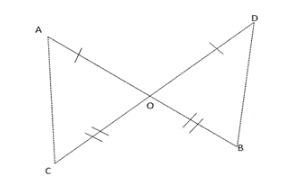Solution:

Given: Line segments AB and CD intersect at O such that OA = OD and OB = OC.

To prove: AC = BD

Proof: In $\Delta$AOC and $\Delta$BOD, we have

AO = OD [Given]

$\angle$AOC  = $\angle$BOD [Vertically opposite angles are equal]

OC = OB [Given]

So, by Side-Angle-Side criterion of congruence, we have,

$\Rightarrow$ AOC $\cong$ $\Delta$BOD

$\Rightarrow$ AC = BD [Since the corresponding parts of the congruent parts of the congruent triangles are equal]

$\Rightarrow$ $\angle$CAO = $\angle$BDO [by c.p.c.t]

Thus, we have, AC = BD

In case   $\angle$ODB = $\angle$OBD, then $\angle$CAO = $\angle$OBD which means alternate angles made by lines AB and BD with transversal AB are equal and then lines AC and BD will be parallel.

Question 3:

In the given figure, l||m and M is the mid-point of AB. Prove that M is also the mid-point of any line segment CD having its end points at l and m respectively.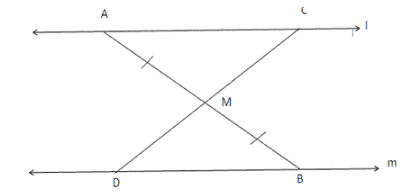Solution:

Given: Two lines l and m are parallel to each other. M is the midpoint of segment AB. The line segment CD meets AB at M.

To prove: M is the midpoint of CD, that is, CM = MD

Proof:

In $\Delta$AMC and $\Delta$BMD, we have

$\angle$MAC = $\angle$MBD [Since l and m are parallel, AB is the transversal, and thus, alternate angles are equal]

AM = MB [Given]

$\angle$AMC = $\angle$BMD [vertically opposite angles are equal]

So, by Angle-Side-Angle criterion of congruence, we have

$\Delta$AMC $\cong$ $\Delta$BMD

Therfore, by corresponding parts of the congruent triangles are equal, we have, CM = MD

Question 4:

In the given figure, AB = AC and OB = OC. Prove that $\angle$ABO = $\angle$ACO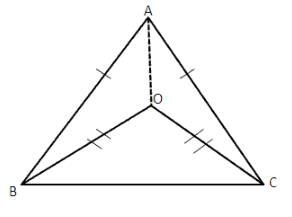Solution:

Given: AB = AC and O is an interior point of the triangle such that OB = OC

To prove: $\angle$ABO = $\angle$ACO

Construction: Join AO

Proof:

In $\Delta$AOB and $\Delta$AOC, we have

AB = AC [Given]

AO = AO [Common]

OB = OC [Given]

So, by Side-Side-Side criterion of congruence, we have,

$\Delta$ABO $\cong$ $\Delta$ACO

$\Rightarrow$ $\angle$ABO = $\angle$ACO [by corresponding parts of congruent triangles are equal]

Question 5:

In the given figure, ABC is a triangle in which AB = AC and D is a point AB. Through D, a line DE is drawn parallel to BC and meeting AC at E. Prove that AD = AE.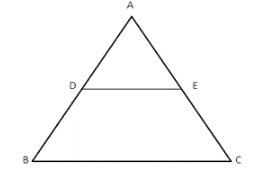Solution:

Given: A $\Delta$ABC in which;

AB = AC

and, DE|| BC

Proof:

Since DE || BC and AB is atransversal.

So, $\angle$ADE = $\angle$ABC —— (i) [These are corresponding angles]

Also DE || BC and AC is a transversal

So, $\angle$AED = $\angle$ACB —— (ii) [These are corresponding angles]

But, AB = AC [Given]

So, $\angle$ABC = ACB ——- (iii)

as opposite angles are also equal in case sides are equal

So from (i), (ii) and (iii) we have

$\angle$ADE = $\angle$AED

and in $\Delta$ADE, this implies that AD = AE.

Question 6:

In the adjoining figure, X and Y are respectively two points on equal sides AB and AC of $\Delta$ABC such that AX = AY. Prove that CX = BY.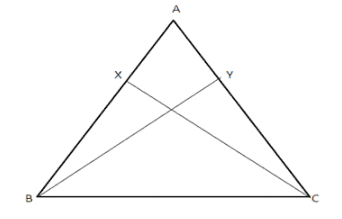Solution:

Given: AX = AY

To prove: CX = BY

Proof: In $\Delta$AXC and $\Delta$AYB, we have

AX = AY [Given]

$\angle$A = $\angle$A [Common angle]

AC = AB [Two sides are equal]

So, by Side-Angle-Side criterion of congruence, we have

$\Delta$AXC $\cong$ $\Delta$AYB

$\Rightarrow$ XC = YB [Since corresponding parts of congruent triangles are equal]

Question 7:

In the given figure, C is the mid-point of AB. If $\angle$DCA = $\angle$ECB and $\angle$DBC = $\angle$EAC, prove that DC = EC.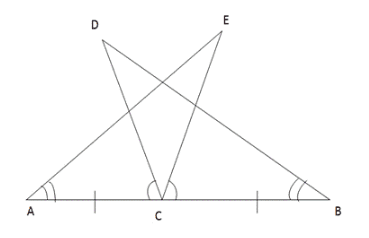Solution:

Given: C is the mid-point of a line segment AB, and D is point such that,

$\angle$DCA = $\angle$ECB

and $\angle$DBC = $\angle$EAC

To prove: DC = EC

Proof:

In $\Delta$ACE and $\Delta$DCB we have;

AC = BC [Given]

$\angle$EAC = $\angle$DBC [Given]

Also, $\angle$DCA = $\angle$CDB + $\angle$DBA because exterior $\angle$DCA in $\Delta$DCB is equal to sum of interior opposite angles.

Again in $\angle$ACE, we have

ext. $\angle$BCE = $\angle$CAE + $\angle$AEC

But, $\angle$DCA = $\angle$BCE [Given]

$\Rightarrow$ $\angle$CDB + $\angle$DBA = $\angle$CAE + $\angle$AEC

$\Rightarrow$ $\angle$CDB = $\angle$AEC [$\angle$DBA = $\angle$CAE (Given)]

Thus in $\Delta$ACE and $\Delta$DCB,

$\angle$EAC = $\angle$DBC

AC = BC

and, $\angle$AEC = $\angle$CDB

Thus by Angle-Side-Angle criterion of congruence, we have

$\Delta$ACE $\cong$ $\Delta$DCB

The corresponding parts of the congruent triangles are equal.

So, DC = CE [by c.c.p.c.t]

Question 8:

In the given figure, BA $\perp$ AC and DE $\perp$ EF such that BA = DE and BF = DC. Prove that AC = EF.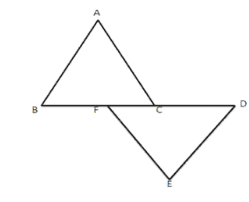Solution:

Given: AB $\perp$ AC and DE $\perp$ FE such that,

AB = DE and BF = CD

To prove: AC = EF

Proof:

In $\Delta$ABC, we have,

BC = BF + FC

and, in $\Delta$DEF

FD = FC + CD

But, BF = CD [Given]

So, BC = BF + FC

and, FD = FC + BF

$\Rightarrow$ BC = FD

So, in $\Delta$ABC and $\Delta$DEF, we have,

$\angle$BAC = $\angle$DEF = 90o [Given]

BC = FD [Proved above]

AB = DE [Given]

Thus, by Right angle-Hypotenuse-Side criterion of congruence, we have

$\Delta$ABC $\cong$ $\Delta$DEF

The corresponding parts of the congruent triangle are equal.

So, AC = EF [c.p.c.t]

AE = $\angle$BCD [Proved above]

Thus by Angle-Side-Angle criterion of congruence, we have

$\Delta$BCD $\cong$ $\Delta$BBAE

The corresponding parts of the congruent triangles are equal.

So, CD = AE [Proved]

Practise This Question

Preeti told Pavani that if the alternate interior angles are equal then lines need not be parallel. Is Preeti's statement true or false?